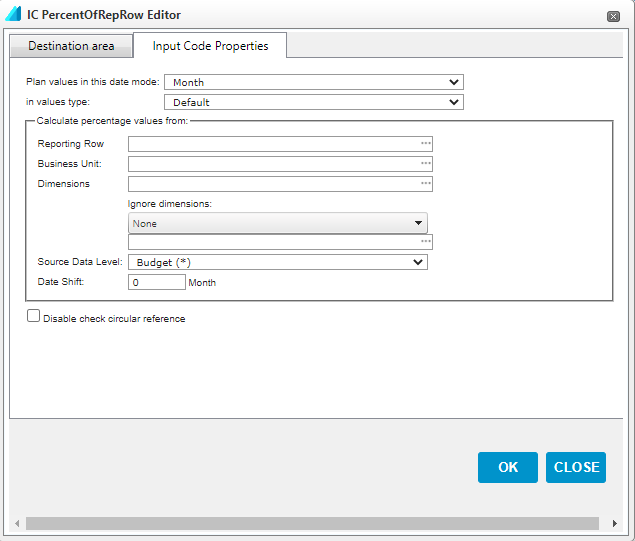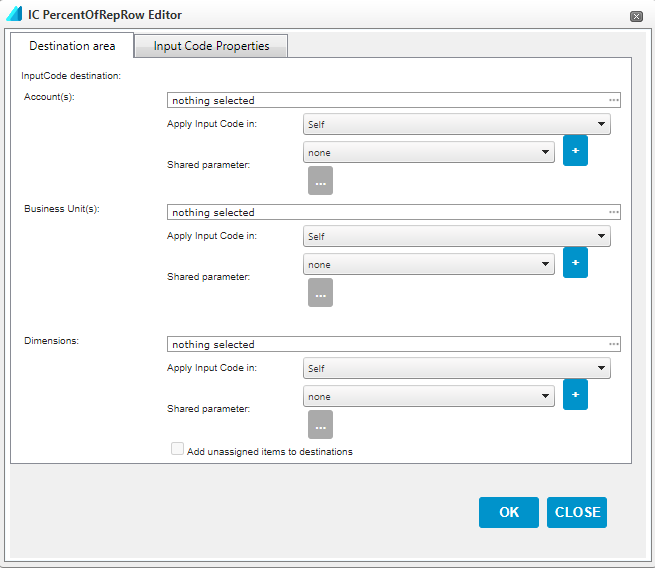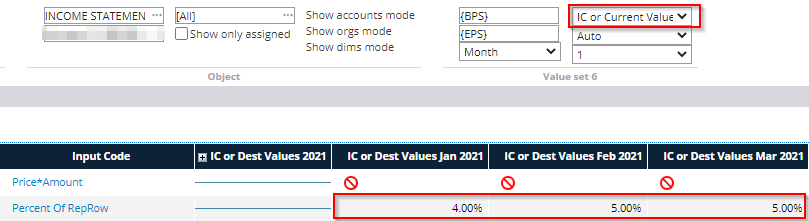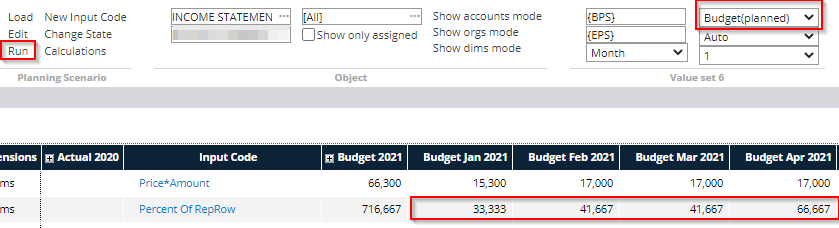Percent of Reporting Row input code can be used to determine that a selected item is a certain percentage of the chosen report row and business unit.

Input Code Properties -tabPlan values in this date mode: This setting determines the accuracy level at which the calculation is performed. With monthly accuracy, a separate percentage is determined for each month, while, for example, with annual accuracy, only one percentage value is determined for the entire year.

In values type: Specifies the type of calculated values stored in the system. In most cases, you can use the default setting, in which case the type of values calculated depends on the settings made on the chart of accounts.

Reporting Row: The Reporting row from which the percentage is calculated.

Business Unit: The organizational level from which the percentage is calculated. If left blank, the source level is the organizational level for which the input code was created.

Source Data Level: The data level from which the percentage is calculated. Options can be, for example, actual, budget or forecast.

Date Shift: A negative value means that the values of the previous month are used as input data, while a positive value means that the percentage is calculated from the values of the following month.

Disable check circular reference: Allows the use of circular references in the input code, i.e. references that depend on the results of the same formula.

Destination area -tabAccount(s): Select the accounts / account groups for which the input code is used

Business Unit(s): The organizational level at which the input code is used

Dimensions(s): Dimensions or dimension objects for which the input code is used. By selecting 'Add unassigned items to destinations', the calculation is also performed for values that are not dimensioned

For all of the above parameters, there are two choices to determine how extensively the calculation is performed:

Apply Input Code in: Specifies whether the input code is used only at the selected level, its cost centers, or parallel levels

Shared parameter: A common parameter that contains user-defined organizational levels or accounts  can be selected in this field. This function can be used if you want to add several different input codes to the same set of accounts or organizations, without defining them in each input code separately. A new common parameter is created from the + sign.

Once all the above parameters have been defined, select 'OK' to create the input code.

Entering the input value and viewing the results

After setting the input code properties, a percentage, ie. the input value, must be set as the basis for the calculation. The input value can only be entered in the 'IC or Current Value' mode, which must be selected from the toolbar as shown below.

The percentage is entered either in the year column or for individual months, depending on the Plan values in this date mode setting in the properties.

In the example, the planning accuracy is set to month, in which case the percentage is entered for each month separately. The annual column has a blue line to indicate that a percentage value cannot be entered on an annual basis.Once the percentages have been entered, the actual calculation for the input form is performed by selecting 'Run' from the toolbar.

The values calculated with the input code can be viewed by selecting Budget or Planned as the display mode, depending on whether the input form is for Budget or Forecast.Once the percentages have been entered, the actual calculation for the input form is performed by selecting 'Run' from the toolbar.